# MATHEMATICS —2004 (Set I— Outside Delhi)

SECTION - A

Q. 1. Solve the following system of linear equations: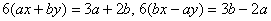Ans.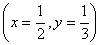Or

The sum of the digits of a two digit number is 15. The number obtained by interchanging the digits exceeds the given number by 9. Find the number.

Ans.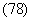Q. 2. Using quadratic formula, solve the following quadratic equation forAns.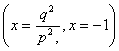Q. 3. Ifis the GCD of the polynomials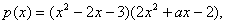and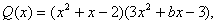find the value of a and b.

Ans.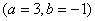Q. 4. If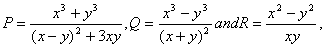Express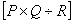as a rational expression in the lowest form.

Ans.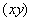Q. 5. The 8 th term of an Arithmetic Progression (A.P.) is 37 and its 12 th term is 57. Find the A.P.

Ans.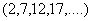Q. 6. Find the sum of the first 25 terms of an A.P. whose n th term is given byAns.Or

Which term of the Arithmetic Progression 3, 10, 17, ... will be 84 more than its 13 th term?

Ans.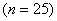Q. 7. An electric fan is available for Rs.600 cash or for Rs.250 cash down payment followed by 3 monthly instalments of Rs.125 each. Find the rate of interest charged under the instalment plan.

Ans.(44.4%)

Q. 8. A loan of Rs 4,200 is to be returned in two equal annual instalments. If the rate of interest is 10% per annum, compounded annually, calculate the amount of each instalment.

Ans. (Rs.2,420)

Q. 9. In Fig. 1, ABCD is a trapezium in which AB || DC. The diagonals AC and BD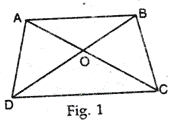intersect at O, Prove thatQ. 10. In Fig. 2, AB is a diameter of a circle, with centre O. If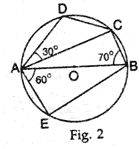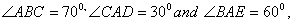findAns.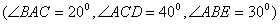SECTION B

Q. 11. Solve the following system of linear equations graphically:Also find the vertices of the triangle formed by the above two lines and x-axis.

Ans.Q.12. Solve for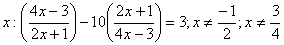Ans.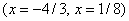Or

300 apples are distributed equally among a certain number of students. Had there been 10 more students, each would have received one apple less. Find the number of students.

Q. 13. A hemispherical bowl of internal diameter 30cm contains some liquid. This liquid is to be filled into cylindrical shaped bottles each of diameter 5cm and height 6cm. Find the number of bottles necessary to empty the bowl.

Ans. (60 bottles)

Q. 14. Prove that :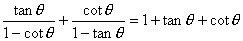Ans.(R.H.S)

Or

Without using trigonometric tables, evaluate the following: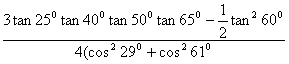Ans.(3/8)

Q. 15. Construct a triangle ABC in which BC = 6.5 cm,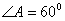and altitude AD = 4.5 cm.

Q. 16. Show that the points A(2, -2), B(14, 10), C(11,13) and D(-1,1) are the vertices of a rectangle.

Q. 17. Prove that the coordinates of the centroid of a, with vertices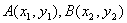andare given by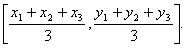Q. 18. The mean of the following frequency distribution is 57.6 and the sum of the observations is 50. Find the missing frequency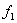and.

Ans.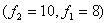Class 0-20 20-40 40-60 60-80 80-100 100-120 Frequency 712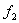8 5

Q. 19. A bag contains 4 red, 5 black and 6 white balls. A ball is drawn from the bag at random. Find the probability that the ball drawn is (i) white (ii) red (iii) not black (iv) red or white.

Ans.(2/5,4/15,2/3,2/3)Q. 20. The following data shows the expenditure of an individual over various items:

 Items Education Food Rent Clothing Others Expenditure 1600 3200 4000 2400 3200

Represent the above data with the help of a pie-chart.

SECTION C

Q. 21. Prove that in a right-angled triangle, the square on the hypotenuse is equal to the sum of the squares on the other two sides.

Using the above, prove the following:

In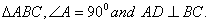Prove that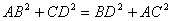Q. 22. Prove that the angle subtended by an arc at the centre is double the angle subtended by it at any point on the remaining part of the circle.

Using the above, do the following:

Prove that the angle formed by a chord in the major segment is acute.

Or

Prove that the sum of either pair of opposite angles of a cyclic quadrilateral is 180 0 . Using the above, do the following:

In Fig. 3, ABCD is a cyclic quadrilateral in which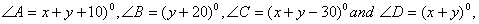Find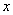and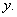Q. 23. If the radii of the circular ends of a bucket, 45 cm high, are 28 cm and 7 cm (as shown in Fig. 4), find the capacity of the bucket.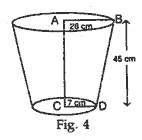Or

A hollow cone is cut by a plane parallel to the base and the upper portion is removed. If the curved surface of the remainder is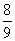of the curved surface of the whole cone, find the ratio of the line segments into which the altitude of the cone is divided by the plane.

Ans. (AB : BD=1 : 2)

Q. 24. A man standing on the deck of a ship, which is 10 m above water level, observes the angle of elevation of the top of a hill as 60° and angle of depression of the base of the hill as 30°. Find the distance of the hill from the ship and height of the hill.

Ans. (distance=17.3 m, height=40 m)

Q. 25. Mohan Lal has an annual income of Rs.2,50,000 (excluding HRA). He contributes Rs. 4,000 per month in his Provident Fund and pays an annual premium of Rs. 15,000 for his LIC policy. How much should he invest in N.S.Cs so as to get maximum rebate? He pays Rs. 2,500 per month for 11 months towards income tax. How much income tax has he to pay in the last month of the year?

Assume the following for calculating income tax:

 (a) Standard Deduction Gross income Deduction (i) Upto Rs.75,000 (ii) From Rs.75,001 to Rs.5,00,000 (iii) More than Rs,5,00,000 40% of gross income Rs.30,000 Rs.20,000 (b) Rates of Income Taxes Income Income tax (i) Upto Rs. 50,000 (ii) From Rs. 50,001 to Rs. 60,000 (iii) From Rs. 60,001 to Rs. 1,50,000 (iv) Rs. 1.50,000 and above No tax 10% of the amount exceeding Rs.50,000 Rs.1,000+20% of the amount by which taxable income exceeds Rs.60,000 Rs.19,000+30% of the amount by which taxable income exceeds Rs.1,50,000 (c) Rebate in Tax (i) 20% of the savings subject to a maximum of Rs. 14,000 if the gross income is upto Rs. 1,50,000. (ii)15% of the savings subject to a maximum of Rs. 10,500 if the gross income is from Rs. 1,50,001 to Rs. 5,00,000.

Ans.(Rs.3,050)

Maths 2004 Question Papers Class X
CBSE 2004 Question Papers Class X